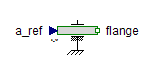Multiphase Multi-Sensor - MapleSim Help

Multi Sensor

Multiphase sensor to measure current, voltage and powerDescription The Multi Sensor component measures currents, voltages, and instantaneous electrical power of a multiphase system, using separate voltage and current paths. The plugs of the voltage paths are pv and nv, the plugs of the current paths are pc and nc. The internal resistance of each current path is zero, the internal resistance of each voltage path is infinite.Equations $i={i}_{\mathrm{pc}}=-{i}_{\mathrm{nc}}$ ${i}_{\mathrm{pv}}={i}_{\mathrm{nv}}=0$ $v={v}_{\mathrm{pv}}-{v}_{\mathrm{nv}}$ ${v}_{\mathrm{pc}}={v}_{\mathrm{nc}}$ ${\mathrm{power}}_{k}={v}_{k}\cdot {i}_{k}$ $\mathrm{powerTotal}=\sum _{k=1}^{m}{\mathrm{power}}_{k}$Connections

 Name Description Modelica ID $\mathrm{pc}$ Positive plug, current path pc $\mathrm{nc}$ Negative plug, current path nc $\mathrm{pv}$ Positive plug, voltage path pv $\mathrm{nv}$ Negative plug, voltage path nv $i$ Current as output signal i $v$ Voltage as output signal v $\mathrm{power}$ Instantaneous power as output signal power $\mathrm{powerTotal}$ Sum of instantaneous power as output signal powerTotalParameters

 Name Default Units Description Modelica ID $m$ $3$ Number of phases mModelica Standard Library The component described in this topic is from the Modelica Standard Library. To view the original documentation, which includes author and copyright information, click here.